# Introduction

The slides of this lecture can be find here

## Stepwise analyses

4. Check your model fit. Are the statistical assumptions met?

5. No, data transformation and corrections

.csv file

``df = read.csv(file = 'name-of-your-file.csv')``

.txt file

``df = read.delim(file = 'name-of-your-file.txt')``

.xlsx file (Excel files also with xls)

``````library(readxl)

df = read_xlsx(path = 'name-of-your-file.xlsx', sheet = 'name of the sheet')``````

``View(df)``

Explore the structure:

``str(df)``
``````## 'data.frame':    180 obs. of  6 variables:
##  \$ TSP          : chr  "1-E34" "10-G17" "100-Q21" "101-Q21" ...
##  \$ C.loss_Mi1   : num  88.3 56.9 86.8 59.4 59.1 ...
##  \$ N.loss_Mi1   : num  96 55 84.1 58.7 68.3 ...
##  \$ lit.rich     : int  1 1 2 1 1 2 1 1 2 2 ...
##  \$ neigh.sp.rich: int  1 1 2 2 2 2 3 1 2 2 ...
##  \$ fall         : num  74 21.8 72 38.2 66.1 ...``````

### 1.3.1. Plot all the variables

``plot(df)``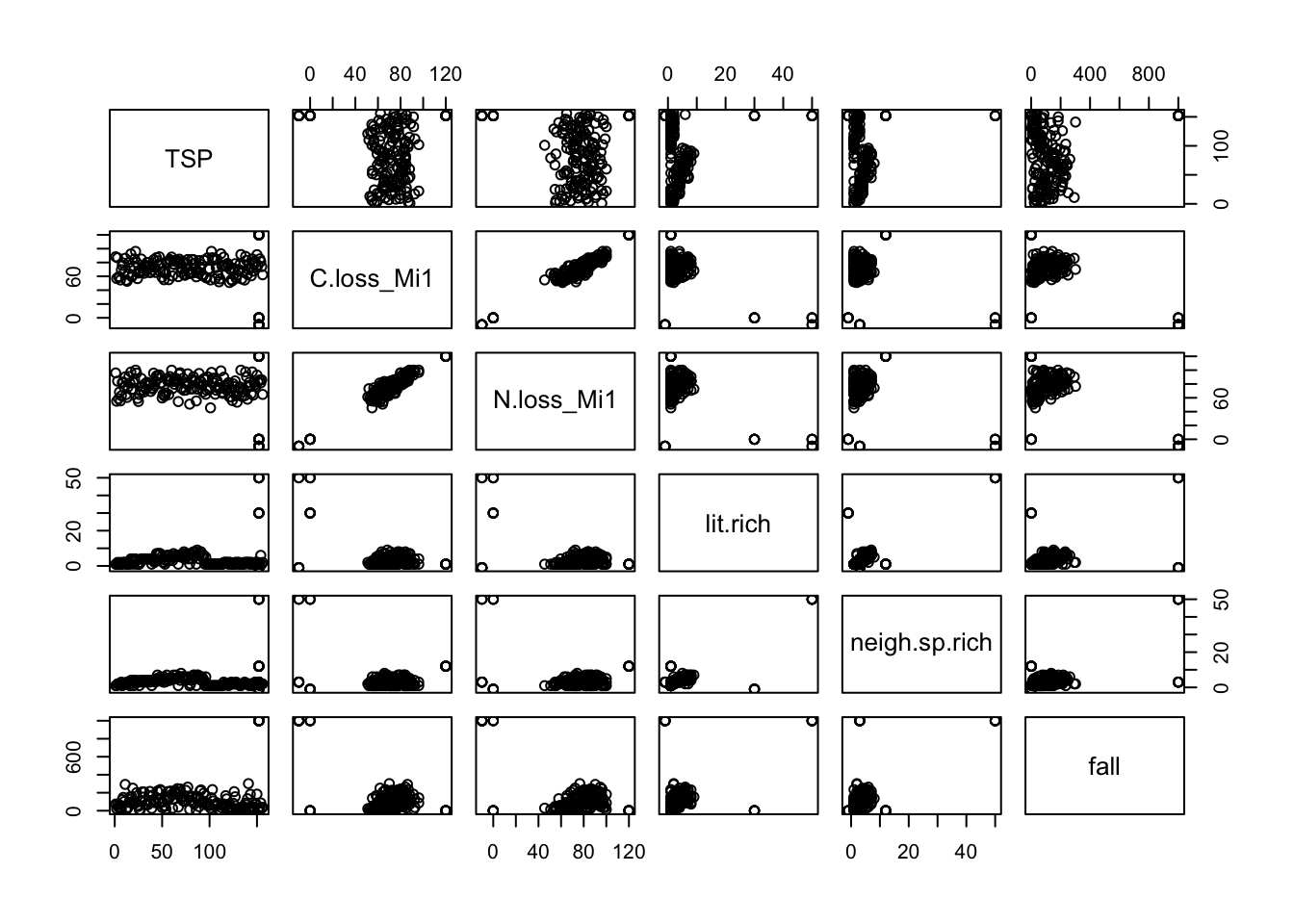### 1.3.2. Plot the variables’ distribution

Example with C loss during decomposition (“C.loss_Mi1”)

``boxplot(df\$C.loss_Mi1)``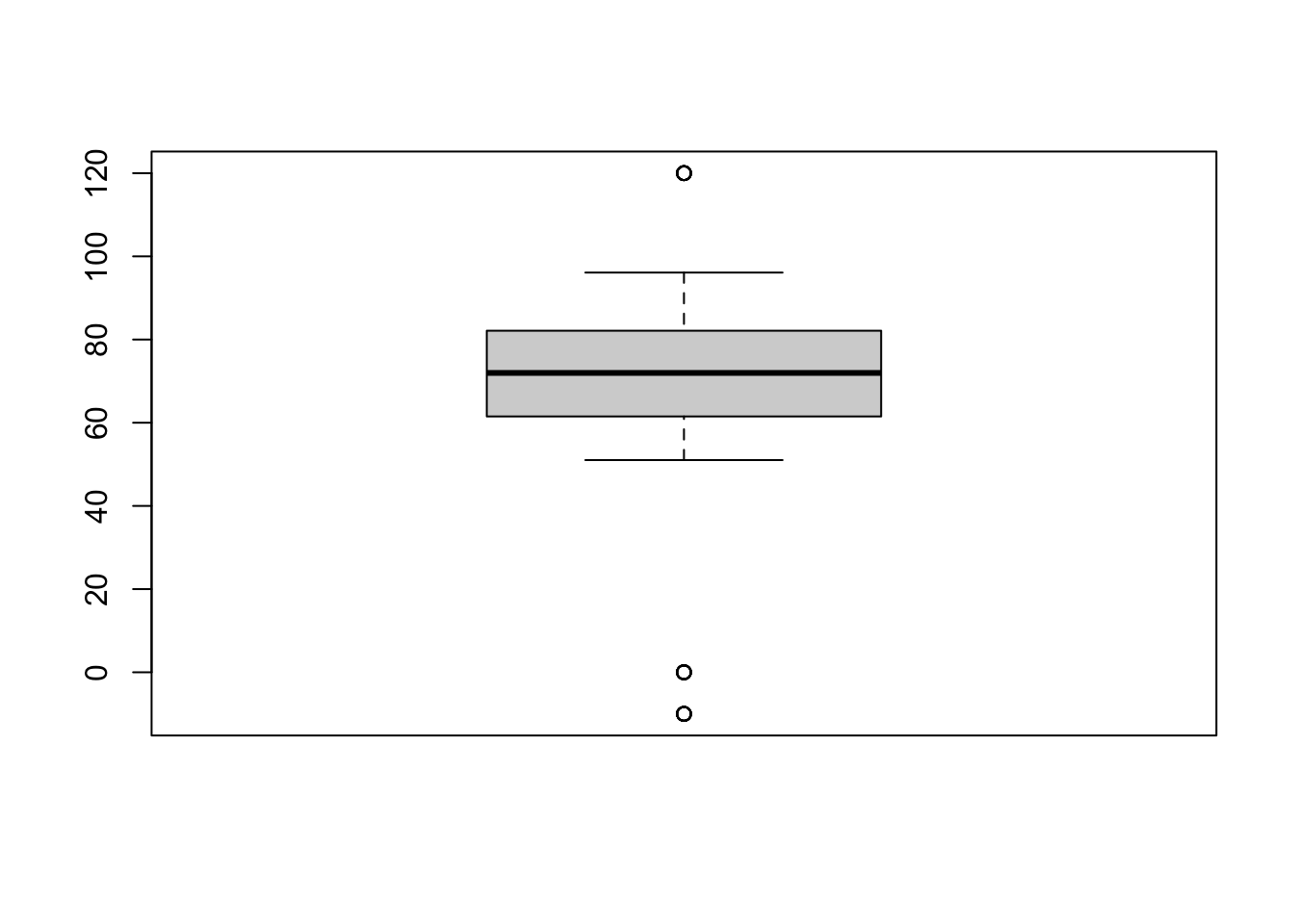ggplot2 version

``````library(ggplot2)

ggplot(data = df, aes(y = C.loss_Mi1, x = " ")) + # structure of the graph
geom_boxplot() +                                # add the boxplot
geom_jitter() +                                 # show the points distribution
labs(x = '', y = "C loss [%]") +                # add labels to the axis
theme_classic()                                 # make it pretty``````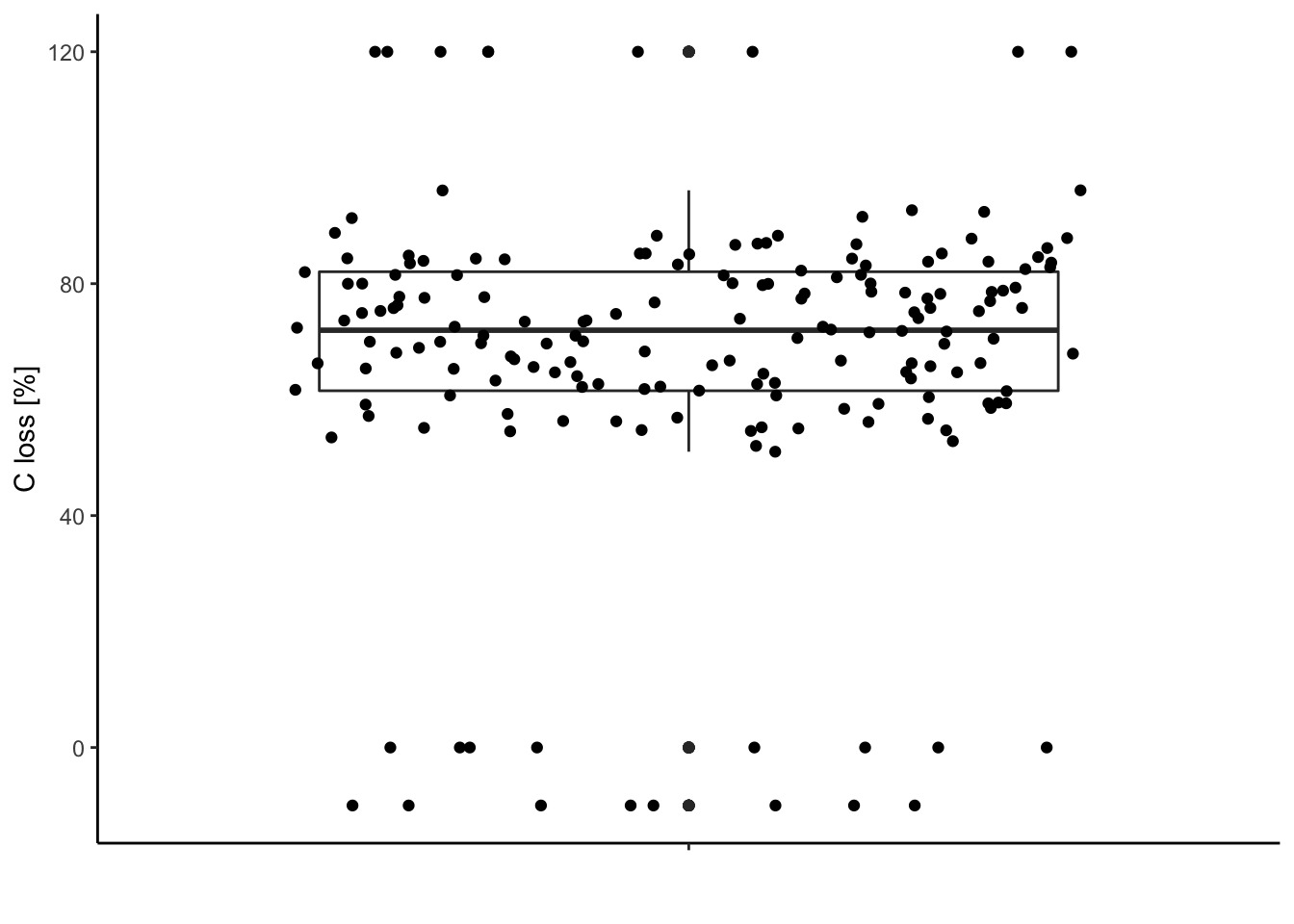### 1.3.3. Compare this distribution to the possible range of data

The carbon loss (%) during litter decomposition should be above 0% (intact leaves) and below 100% (completely decomposed).

Check for data out of the range (possible typos, mistakes during measurements …).

Visually:

``````ggplot(data = df, aes(y = C.loss_Mi1, x = " ")) + # structure of the graph
geom_boxplot() +                                # add the boxplot
geom_jitter() +                                 # show the points distribution
labs(x = '', y = "C loss [%]") +                # add labels to the axis
theme_classic() +                               # make it pretty
geom_hline(yintercept = 0) +                    # add line at 0%
geom_hline(yintercept = 100)                    # add line at 100% ``````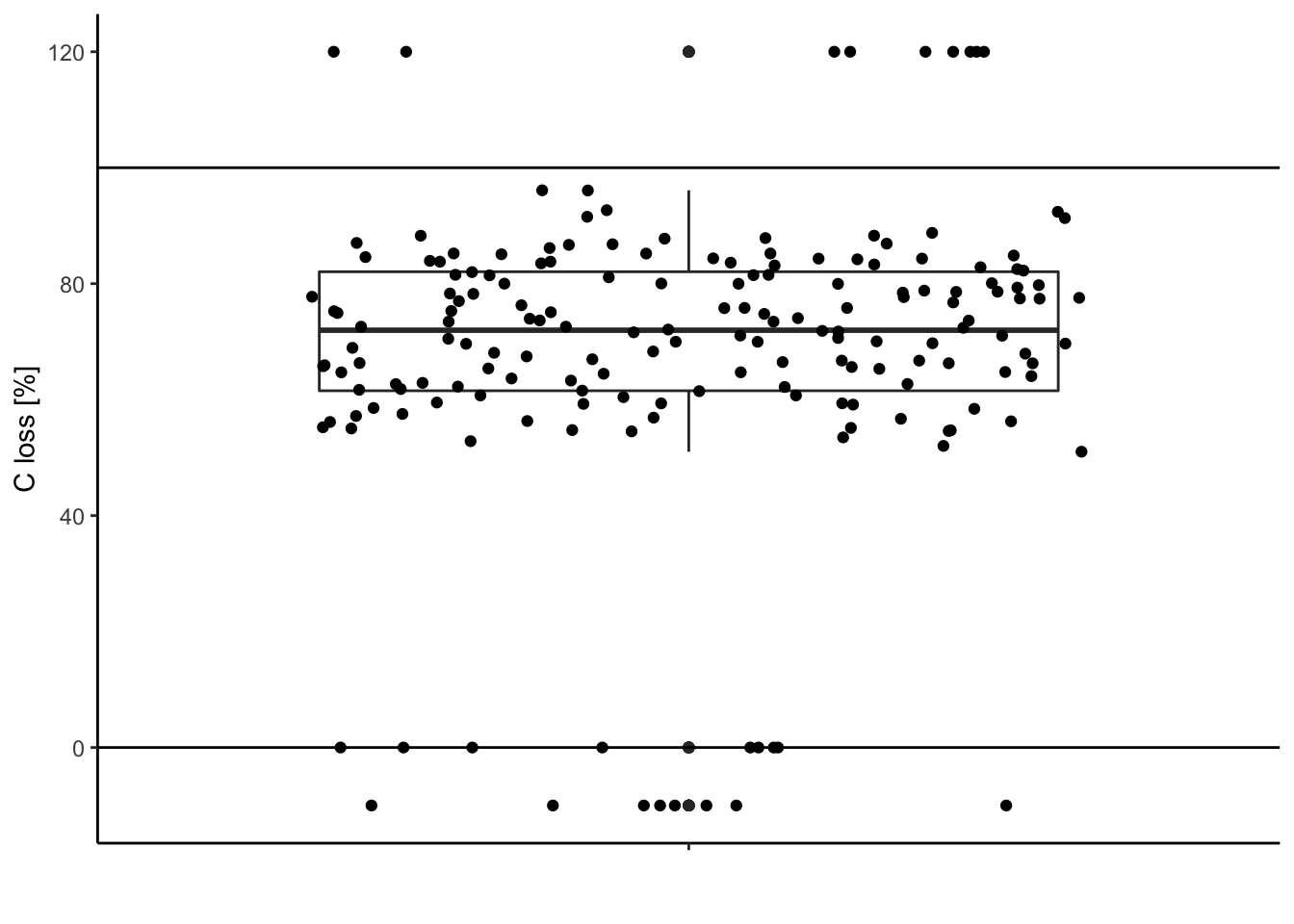Extract these outliers:

``df[df\$C.loss_Mi1 < 0 | df\$C.loss_Mi1 > 100,] # | means "OR"``
``````##          TSP C.loss_Mi1 N.loss_Mi1 lit.rich neigh.sp.rich fall
## 156 outliers        120        120        1            12    1
## 157 outliers        120        120        1            12    1
## 158 outliers        120        120        1            12    1
## 159 outliers        120        120        1            12    1
## 160 outliers        120        120        1            12    1
## 161 outliers        120        120        1            12    1
## 162 outliers        120        120        1            12    1
## 163 outliers        120        120        1            12    1
## 164 outliers        120        120        1            12    1
## 173 outliers        -10        -10       50            50 1000
## 174 outliers        -10        -10       50            50 1000
## 175 outliers        -10        -10       -1             3 1000
## 176 outliers        -10        -10       -1             3 1000
## 177 outliers        -10        -10       -1             3 1000
## 178 outliers        -10        -10       -1             3 1000
## 179 outliers        -10        -10       -1             3 1000
## 180 outliers        -10        -10       -1             3 1000``````

With dplyr:

``````library(dplyr)

df %>%
filter(C.loss_Mi1 < 0 | C.loss_Mi1 > 100) # filter your rows under conditions``````
``````##         TSP C.loss_Mi1 N.loss_Mi1 lit.rich neigh.sp.rich fall
## 1  outliers        120        120        1            12    1
## 2  outliers        120        120        1            12    1
## 3  outliers        120        120        1            12    1
## 4  outliers        120        120        1            12    1
## 5  outliers        120        120        1            12    1
## 6  outliers        120        120        1            12    1
## 7  outliers        120        120        1            12    1
## 8  outliers        120        120        1            12    1
## 9  outliers        120        120        1            12    1
## 10 outliers        -10        -10       50            50 1000
## 11 outliers        -10        -10       50            50 1000
## 12 outliers        -10        -10       -1             3 1000
## 13 outliers        -10        -10       -1             3 1000
## 14 outliers        -10        -10       -1             3 1000
## 15 outliers        -10        -10       -1             3 1000
## 16 outliers        -10        -10       -1             3 1000
## 17 outliers        -10        -10       -1             3 1000``````

You can inverse the condition and save the selected rows in df. Be careful, this will overwrite your data frame (in R), it is always wise to have a save copy.

``````df.save = df # save the data before cleaning in df.save

df =
df %>%
filter(!(C.loss_Mi1 < 0 | C.loss_Mi1 > 100)) # "!" inverse the conditions``````

Alternatives:

``````df =
df %>%
filter(C.loss_Mi1 >= 0 & C.loss_Mi1 <= 100)        # "&" both conditions needed

df = df[df\$C.loss_Mi1 >= 0 & df\$C.loss_Mi1 <= 100, ] # in base R``````

Data after cleaning:

``````ggplot(data = df, aes(y = C.loss_Mi1, x = " ")) + # structure of the graph
geom_boxplot() +                                # add the boxplot
geom_jitter() +                                 # show the points distribution
labs(x = '', y = "C loss [%]") +                # add labels to the axis
theme_classic() +                               # make it pretty
geom_hline(yintercept = 0) +                    # add line at 0%
geom_hline(yintercept = 100)                    # add line at 100% ``````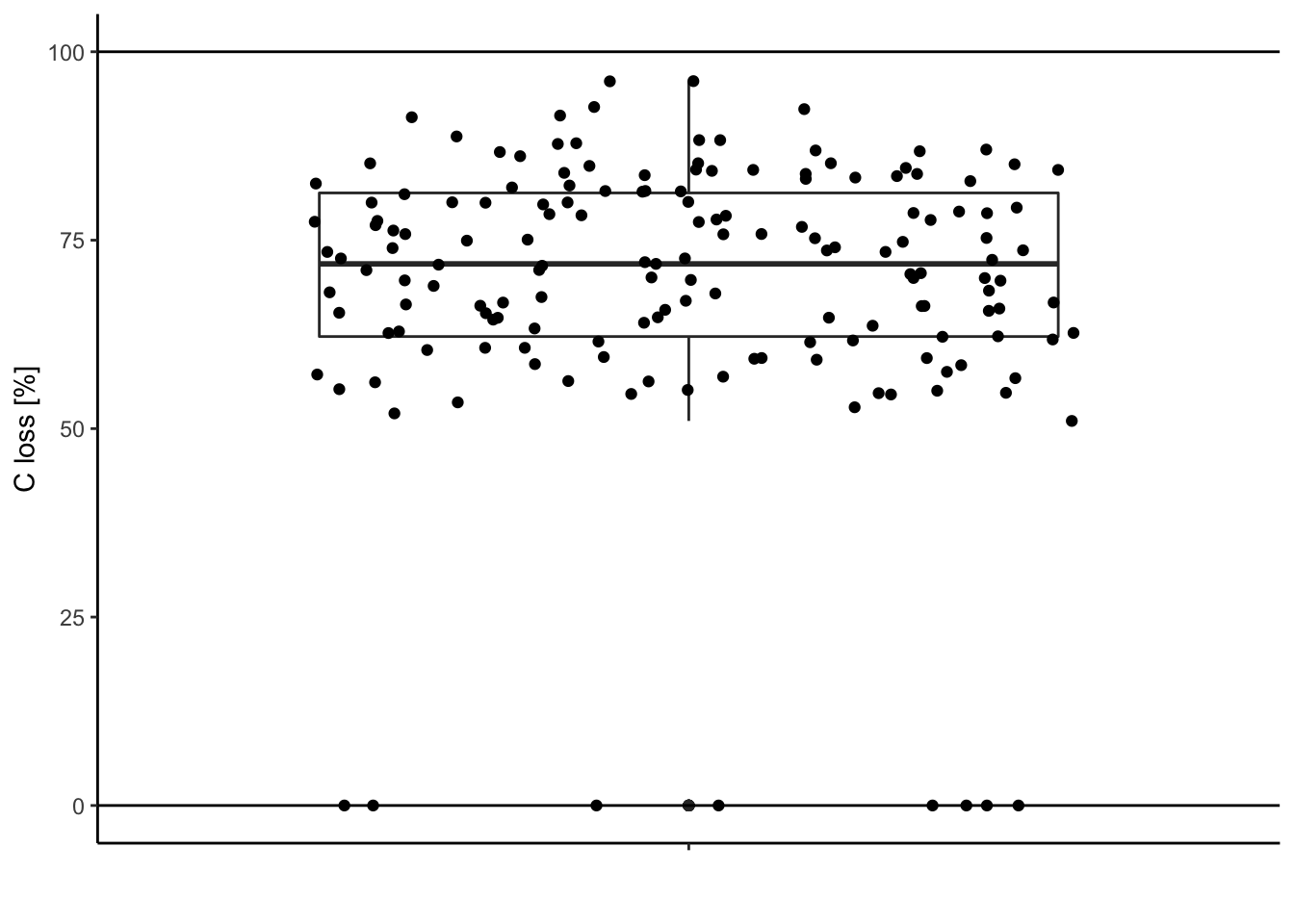Looks better, no?!

## 2.1. Hypotheses

This as nothing to do with R. In our case:

We expect carbon loss (C.loss_Mi1) to increase with litter species richness (lit.rich).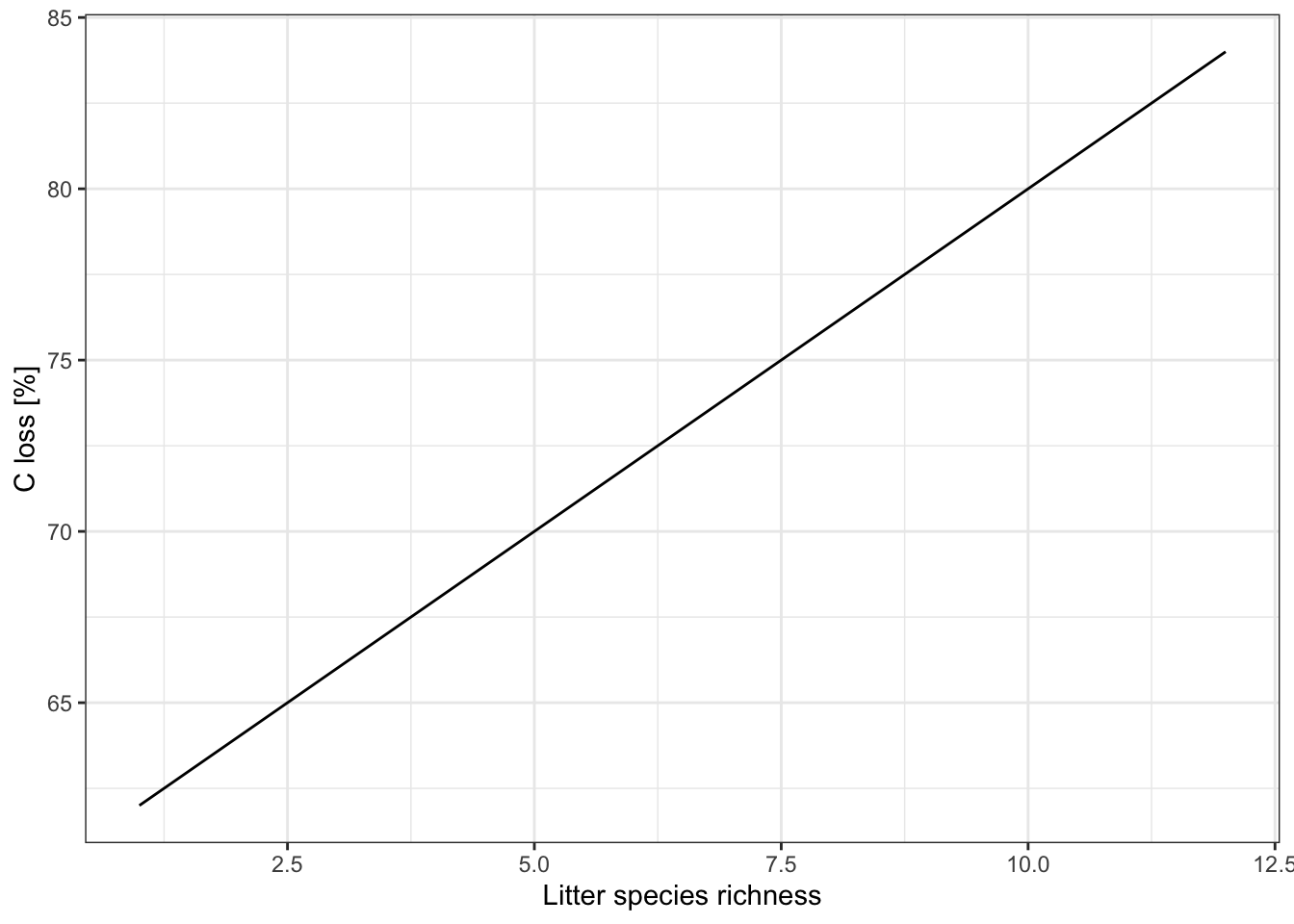## 2.2. distribution

What is the expected distribution of the response variable C.loss?

In short:

DistributionSamplingExample
NormalContinuous measurementssize, flux, biomass …
PoissonDiscrete countingnumber of species, number of individuals
BinomialYes/Nopresence-absence of species
BetaPercentagesdecomposition rate

N.B. the beta distribution takes into account the bounding between 0 and 1 (or 0 and 100). In our case where our variable values are far from these limits, the normal distribution can also be used and will give similar results.

## 3.1. Identify the parameters of your model

Response variable: C.loss_Mi1

Explanatory variable: lit.rich

Formula: C.loss_Mi1 ~ lit.rich

Relation type: linear

Data frame: df

Distribution of the residuals: Normal

``mod = lm(data = df, formula = 'C.loss_Mi1 ~ lit.rich') # the lm function fit linear model with Normal distribution assumption on the residuals``

Other residual distribution type:

Poisson distribution

``mod = glm(data = df, formula = 'y ~ x', family = poisson())``

Binomial distribution

``mod = glm(data = df, formula = 'y ~ x', family = binomial())``

# 4. Check your model fit. Are the statistical assumption met?

New in town: the “performance” package

## 4.1. Model fit

``````library(performance)

check_model(mod)``````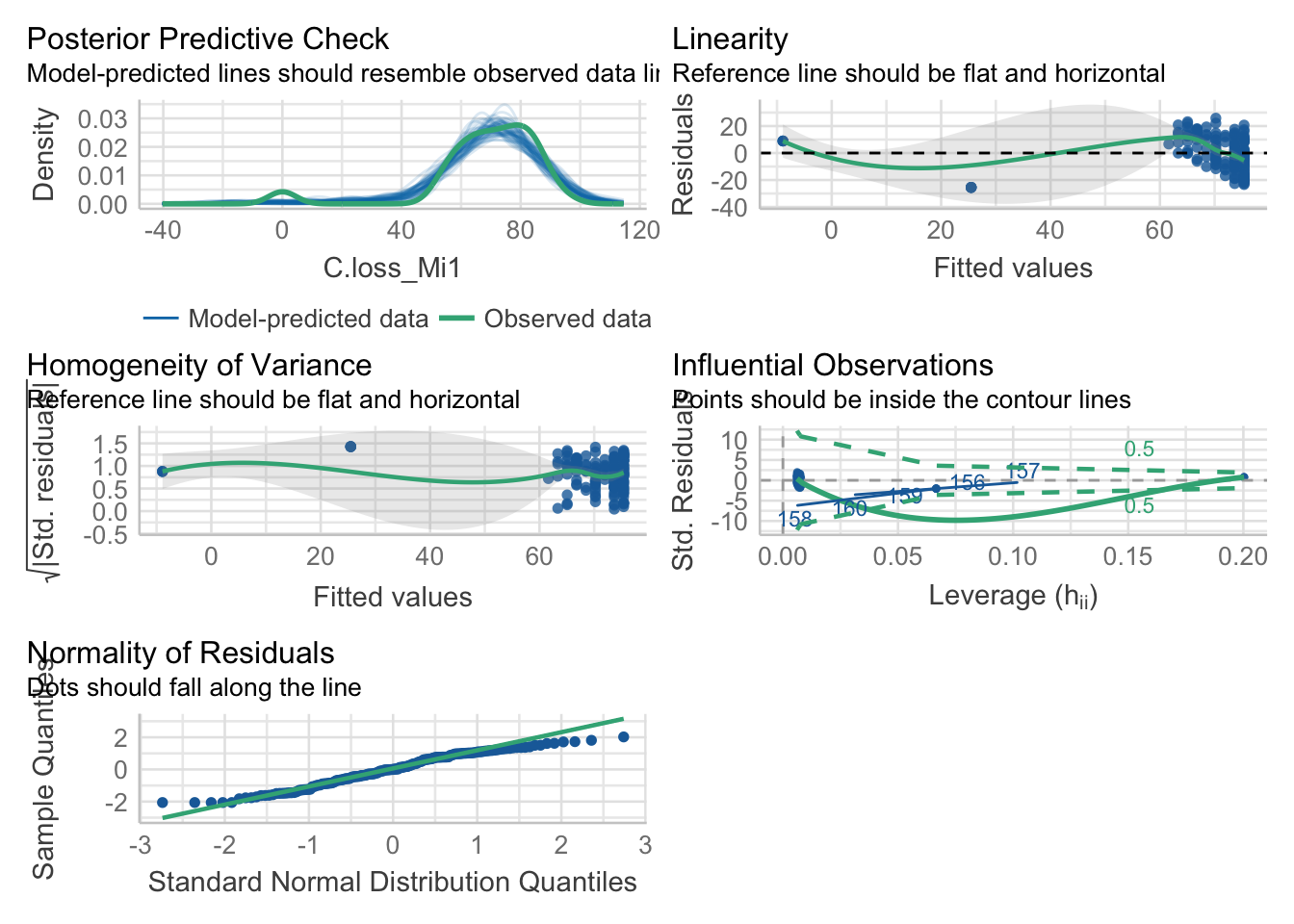## 4.2. Check outliers

``check_outliers(mod)``
``## OK: No outliers detected.``

## 4.3. Check possible distribution of residuals

``check_distribution(mod)``
``````## # Distribution of Model Family
##
## Predicted Distribution of Residuals
##
##  Distribution Probability
##        normal         66%
##       tweedie         22%
##       weibull          6%
##
## Predicted Distribution of Response
##
##                Distribution Probability
##                     tweedie         59%
##  neg. binomial (zero-infl.)          9%
##                      normal          9%``````

## 4.4. Check normality of the residuals

``check_normality(mod)``
``## Warning: Non-normality of residuals detected (p = 0.002).``

## 4.5. Check heteroscedasticity of variance

``check_heteroscedasticity(mod)``
``## Warning: Heteroscedasticity (non-constant error variance) detected (p = 0.021).``

Remember that these tests are often really conservative. They are useful to make your choices but need to be considered having in mind the sample size and data structure.

Here, our model doesn’t seem to meet the assumption of heteroscedasticity and normality of the residuals.

# 5. No, data transformation and corrections

## 5.1. Check your data (again)

Look for surprising values, they could be typos.

``````ggplot(data = df, aes(y = C.loss_Mi1, x = " ")) + # structure of the graph
geom_rect(data = NULL,                          # add rectangle to show outliers
aes(ymin = -5, ymax = 5,
xmin = -Inf, xmax = Inf),
alpha = .005, fill = 'red', color = NA) +
geom_boxplot() +                                # add the boxplot
geom_jitter() +                                 # show the points distribution
labs(x = '', y = "C loss [%]") +                # add labels to the axis
theme_classic() +                               # make it pretty
geom_hline(yintercept = 0) +                    # add line at 0%
geom_hline(yintercept = 100)                  # add line at 100% ``````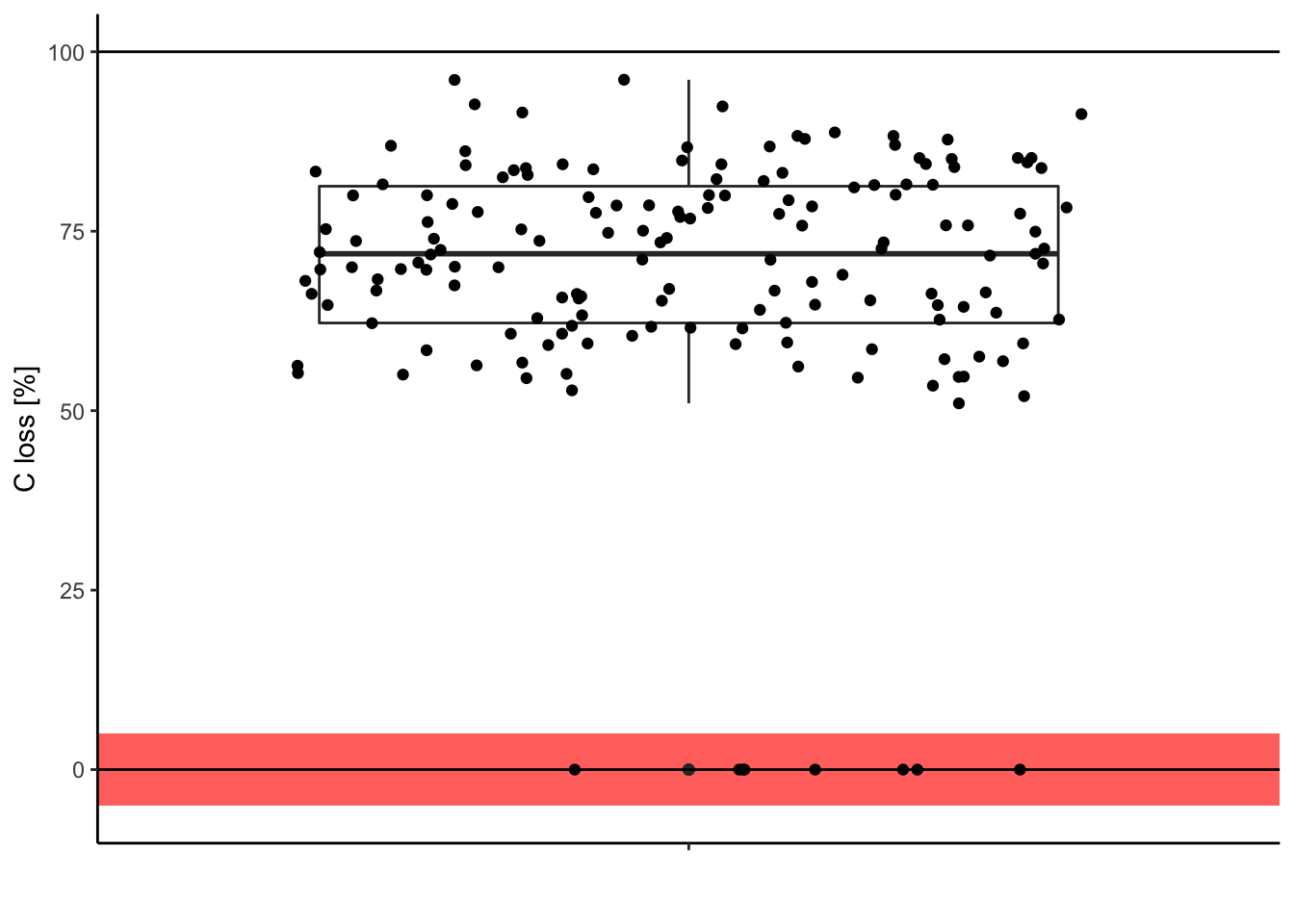Is it meaningful to have 0% of carbon loss after one year of decomposition? In this case, it was only typos. So we can correct them in the original data.

Look at the relationship you are fitting

``````ggplot(data = df, aes(x = lit.rich, y = C.loss_Mi1)) +
geom_jitter() +
# all the regression line using a linear relationship (i.e. here the same that your model)
geom_smooth(method = 'lm', color = 'black') +
labs(x = "Litter species richness", y = "C loss [%]") +
theme_classic()``````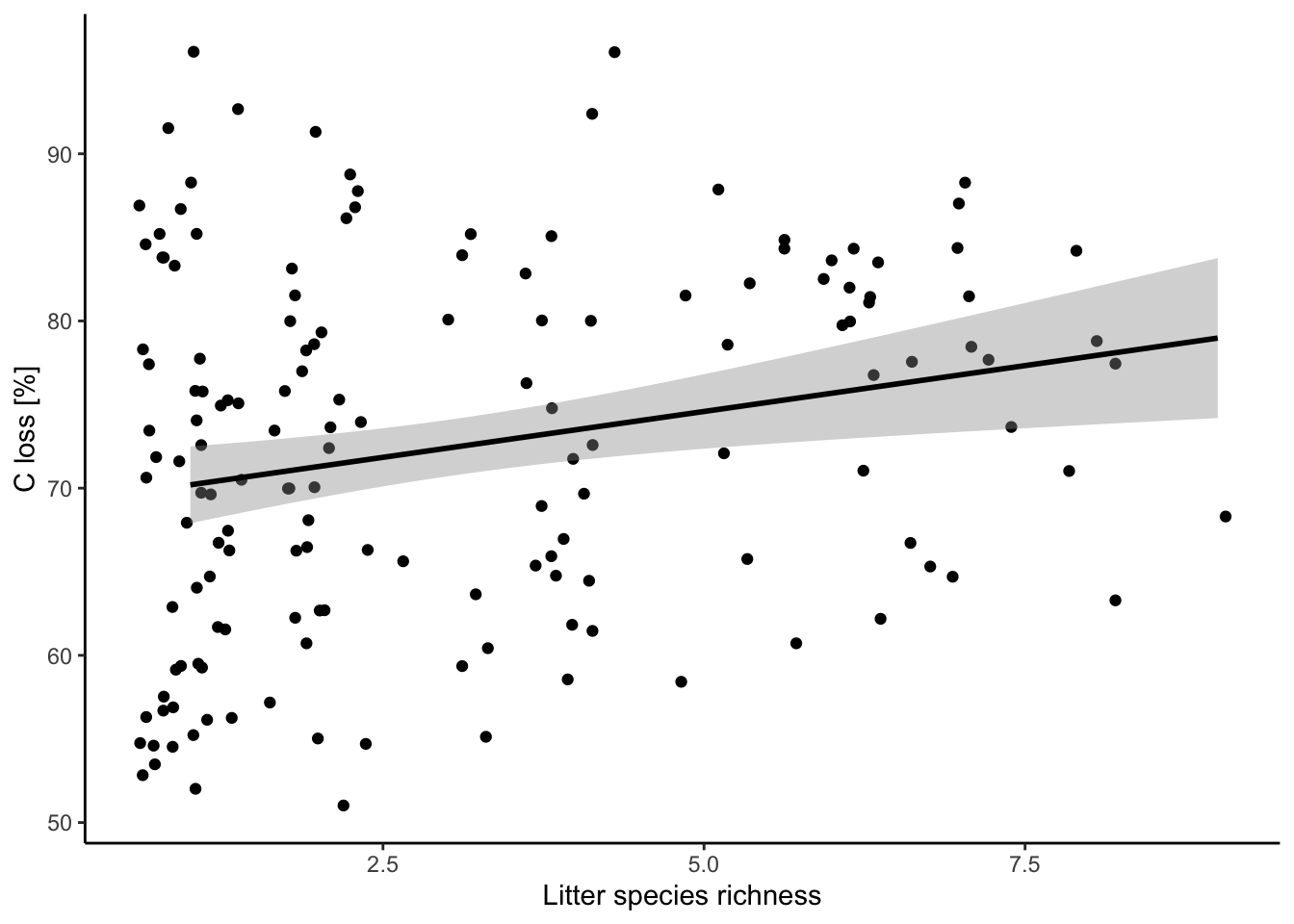## 5.2. New model after corrections

``````mod = lm(data = df, formula = 'C.loss_Mi1 ~ lit.rich')
check_model(mod)``````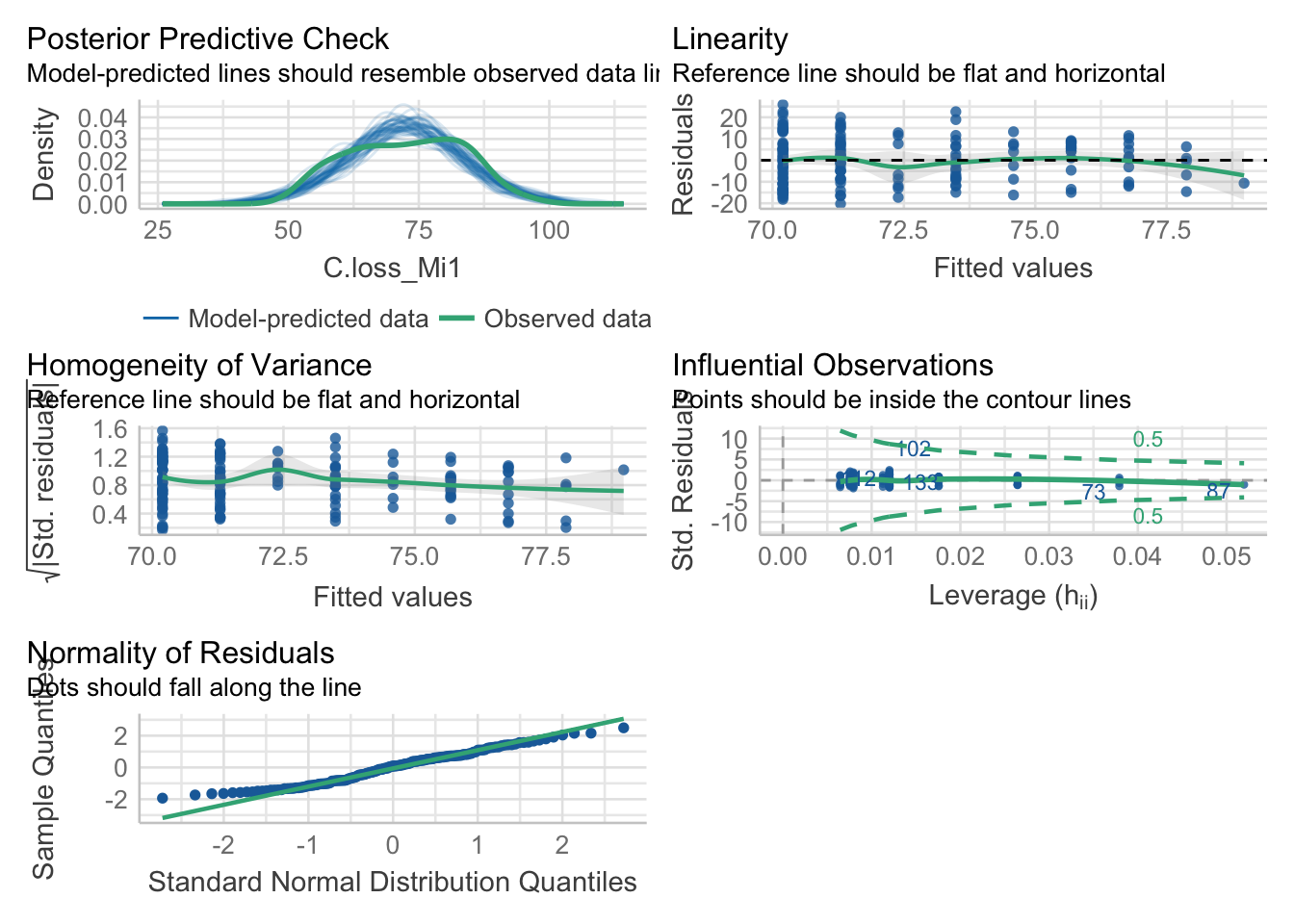## 5.3. Data transformation

Log transformation of your explanatory variable.

``````ggplot(data = df, aes(x = lit.rich, y = C.loss_Mi1)) +
geom_jitter() +
# all the regression line using a linear relationship (i.e. here the same that your model)
geom_smooth(method = 'lm', color = 'black') +
scale_x_continuous(trans = 'log', breaks = c(1,2,4,8)) +
labs(x = "Litter species richness", y = "C loss [%]") +
theme_classic()``````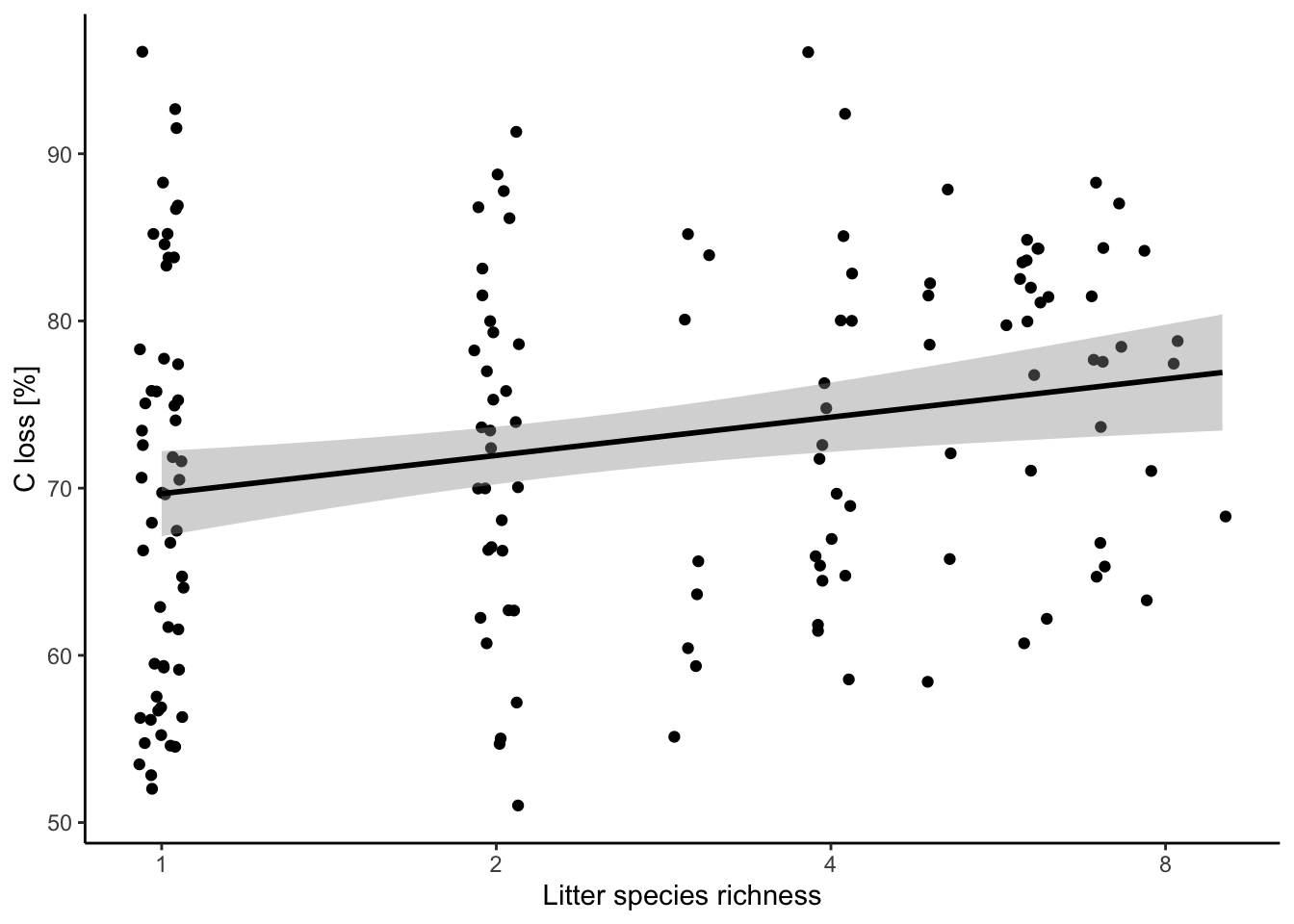New model

``````mod.1 = lm(data = df, formula = 'C.loss_Mi1 ~ log(lit.rich)')

check_model(mod.1)``````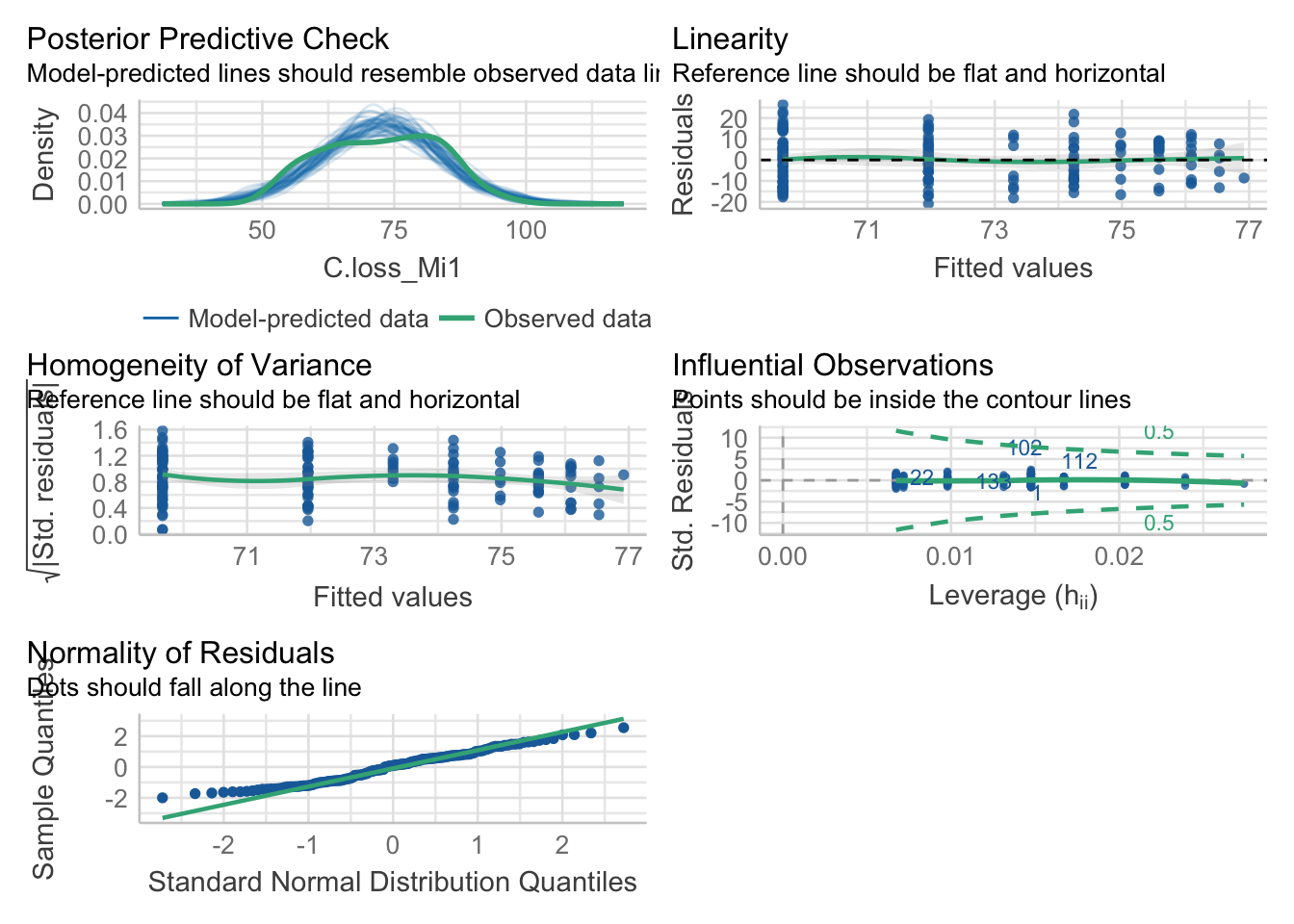## 5.4. Compare the model quality

``compare_performance(mod, mod.1)``
``````## # Comparison of Model Performance Indices
##
## Name  | Model |      AIC | AIC weights |      BIC | BIC weights |    R2 | R2 (adj.) |   RMSE |  Sigma
## -----------------------------------------------------------------------------------------------------
## mod   |    lm | 1176.469 |       0.484 | 1185.599 |       0.484 | 0.051 |     0.045 | 10.557 | 10.626
## mod.1 |    lm | 1176.338 |       0.516 | 1185.469 |       0.516 | 0.052 |     0.046 | 10.552 | 10.621``````

Data transformation doesn’t improve our model

# 6. Yes, extract your results

## 6.1. Check model summary

``summary(mod)``
``````##
## Call:
## lm(formula = "C.loss_Mi1 ~ lit.rich", data = df)
##
## Residuals:
##      Min       1Q   Median       3Q      Max
## -20.2747  -8.7829   0.8984   7.5652  25.9039
##
## Coefficients:
##             Estimate Std. Error t value Pr(>|t|)
## (Intercept)  69.1002     1.4516  47.602  < 2e-16 ***
## lit.rich      1.0972     0.3824   2.869  0.00469 **
## ---
## Signif. codes:  0 '***' 0.001 '**' 0.01 '*' 0.05 '.' 0.1 ' ' 1
##
## Residual standard error: 10.63 on 153 degrees of freedom
## Multiple R-squared:  0.05107,    Adjusted R-squared:  0.04487
## F-statistic: 8.234 on 1 and 153 DF,  p-value: 0.004693``````

## 6.2. Extract model coefficients

``summary(mod)\$coefficients``
``````##              Estimate Std. Error   t value     Pr(>|t|)
## (Intercept) 69.100160  1.4516329 47.601675 1.272742e-93
## lit.rich     1.097198  0.3823676  2.869485 4.693330e-03``````

## 6.3. Extract model R squared

``summary(mod)\$r.squared``
``##  0.05106831``

## 6.4. Extract your fitted model

``````library(ggeffects)

predictions = ggpredict(model = mod, terms = 'lit.rich')

predictions``````
``````## # Predicted values of C.loss_Mi1
##
## lit.rich | Predicted |         95% CI
## -------------------------------------
##        1 |     70.20 | [67.92, 72.48]
##        2 |     71.29 | [69.44, 73.15]
##        3 |     72.39 | [70.72, 74.07]
##        4 |     73.49 | [71.68, 75.30]
##        5 |     74.59 | [72.38, 76.80]
##        6 |     75.68 | [72.92, 78.44]
##        7 |     76.78 | [73.39, 80.17]
##        9 |     78.97 | [74.23, 83.72]``````

``````ggplot(data = df, aes(x = lit.rich, y = C.loss_Mi1)) +         # frame
geom_jitter(data = df, aes(x = lit.rich, y = C.loss_Mi1)) +  # jitter add some noise in the x-axis to better show the points
geom_line(data = predictions, aes(x = x, y = predicted)) +   # add the regression line, when you use different dataframes in the plot you need to define them for each geom
labs(x = "Litter species richness", y = "C loss [%]") +
theme_classic()``````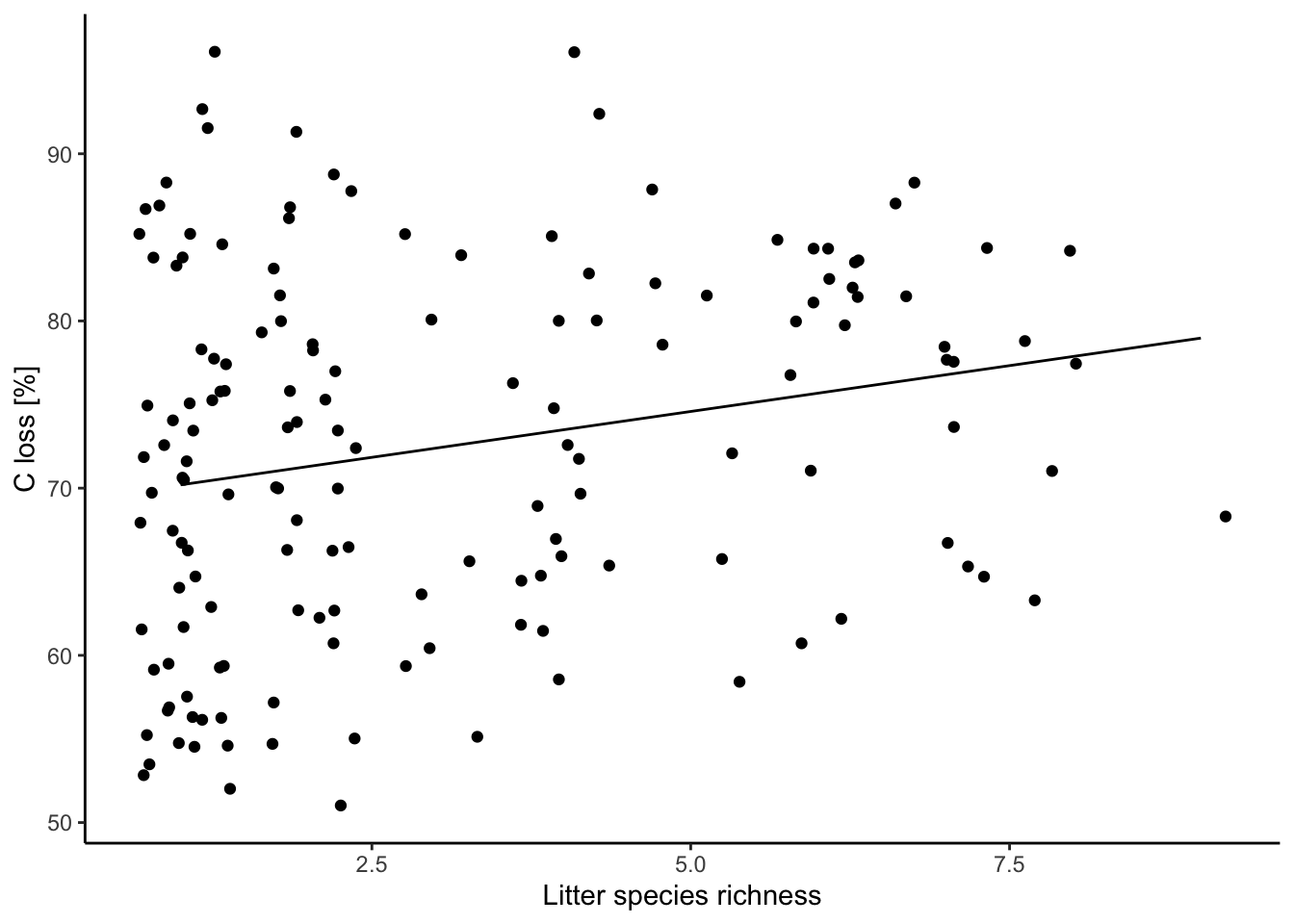See ggplot packages for more details.##### Rémy Beugnon

I am a PosDoc between iDiv, the CEFE and LIM working vegetation diversity effects on soil microclimate.